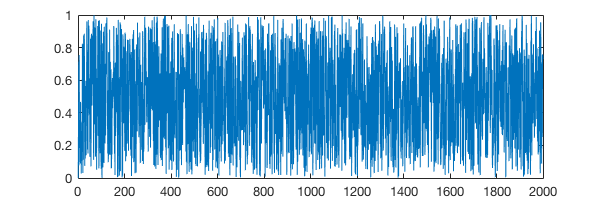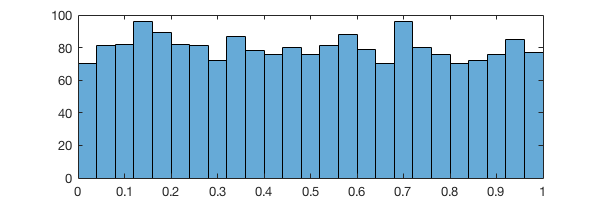# Lecture 4: Floats & Random Numbers¶

Date: 09/12/2017, Tuesday

In :

format compact
format long % print more digits


## Floating point number system¶

Double precision:

$\begin{split}x = \pm(1+f)2^e \\ 0 \le 2^{t}f<2^{t}, t=52 \\ -1022 \le e \le 1023\end{split}$

(See lecture slides or textbook for more explantion. This website focuses on codes.)

How many binary bits are needed to store $$e$$:

In :

log2(2048)

ans =
11


### Maximum value¶

Calculate the maximum value of $$x$$ from the formula.

In :

t=52;
f=(2^t-1)/2^t;
(1+f)*2^1023

ans =
1.797693134862316e+308


Compare with the built-in function

In :

realmax

ans =
1.797693134862316e+308


What happens if the value exceeds realmax?

In :

2e308

ans =
Inf


### Minimum (absolute) value¶

From the formula

In :

2^-1022

ans =
2.225073858507201e-308


Compare with the built-in function

In :

realmin

ans =
2.225073858507201e-308


MATLAB allows you to go lower than realmin, but no too much.

In :

for k=-321:-1:-325
fprintf('k = %d, 10^k = %e \n',k,10^k)
end

k = -321, 10^k = 9.980126e-322
k = -322, 10^k = 9.881313e-323
k = -323, 10^k = 9.881313e-324
k = -324, 10^k = 0.000000e+00
k = -325, 10^k = 0.000000e+00


$$10^{-323}$$ can be scaled up:

In :

1e-323 * 1e300

ans =
9.881312916824931e-24


But $$10^{-324}$$ can’t, as it becomes exactly 0.

In :

1e-324 * 1e300

ans =
0


### Machine precision¶

#### Compute machine precision¶

From the formula $$0 \le 2^{t}f<2^{t}, t=52$$

In :

2^(-52)

ans =
2.220446049250313e-16


Built-in function:

In :

eps

ans =
2.220446049250313e-16


Another ways to get eps

In :

1.0-(0.1+0.1+0.1+0.1+0.1+0.1+0.1+0.1+0.1+0.1) % equals to eps/2

ans =
1.110223024625157e-16

In :

7/3-4/3-1 % equals to eps

ans =
2.220446049250313e-16


#### Difference between eps and realmin¶

realmin is about abosolute magnitude, while eps is about relative accuracy. Although a double-precision number can represent a value as small as $$10^{-323}$$ (i.e. realmin), the relative error of arithmetic operations can be as large as $$10^{-16}$$ (i.e. eps).

Adding $$10^{-16}$$ to 1.0 has no effect at all.

In :

1.0+1e-16-1.0

ans =
0


Adding $$10^{-15}$$ to 1.0 has some effect, although the result is quite inaccurate.

In :

1.0+1e-15-1.0

ans =
1.110223024625157e-15


### Not a number¶

In :

0/0

ans =
NaN

In :

Inf - Inf

ans =
NaN


However, Inf can sometimes be meaningful: (MATLAB-only. Not true in low-level languages.)

In :

5/Inf

ans =
0

In :

5/0

ans =
Inf


## Random numbers¶

Linear congruential generator

In :

a = 22695477;
c = 1;
m = 2^32;
N = 2000;

X = zeros(N,1);
X(1) = 1000;
for j=2:N
X(j)=mod(a*X(j-1)+c,m);
end

R = X/m;


Hmm… looks pretty random🤔

In :

%plot --size 600,200
plot(R);The data also looks like evenly-distributed.

In :

nbins = 25;
histogram(R, nbins);